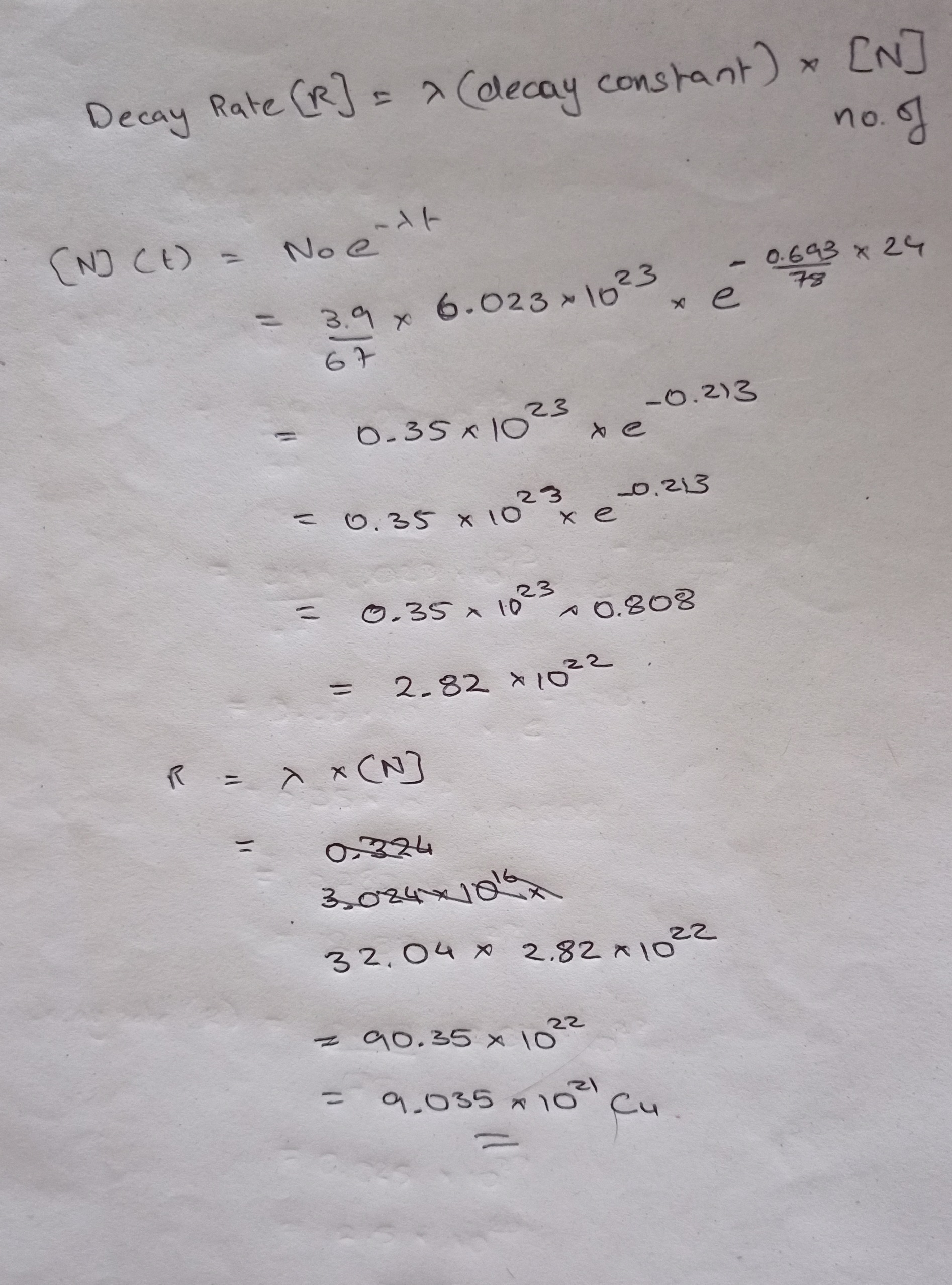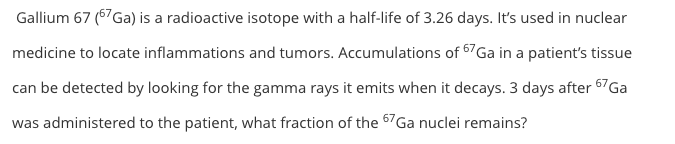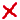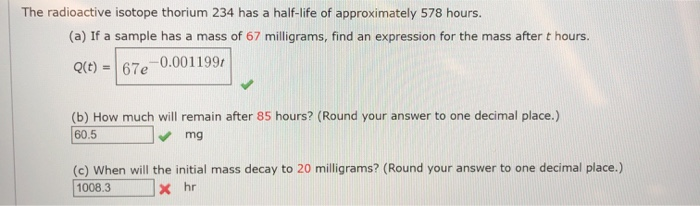# An isotope of gallium, 67Ga, has an atomic number of 31 and a half-life of 78...

An isotope of gallium, 67Ga, has an atomic number of 31 and a half-life of 78 hours. Consider a small mass of 3.9 grams for 67Ga which is initially pure.

1) Initially, what is the half-life of the gallium?

T1/2o =

2) Initially, what is the initial decay constant of the Ga atom in the sample?

λo =

3) Initially, what is the decay rate of the gallium?

Ro =

4) What is the half-life of the gallium after 24 hours?

T1/21 =

5) Initially, what is the initial decay constant of the Ga atoms after 24 hours?

λ1 =

6) What is the decay rate of the gallium after 24 hours?

R1 =

1) Initial t1/2=78hrs

2)initial decay constant=0.693/t1/2

=0.693/78

=0.0089/hr or

​32.04/sec

3)decay rate = decay constant*number of particles

=(0.0089*3.9*6.023*1023)/67

=3.12*1023particles per hr

Or 3.024*1016 Cu

4)Half life remains same after 24 hrs

5)Decay constant remains same after 24 hrs

6)decay rate= decay constant *no. of particles

The math is attatched as an image##### Add Answer of: An isotope of gallium, 67Ga, has an atomic number of 31 and a half-life of 78...
Similar Homework Help Questions
• ### Gallium 67 (67Ga) is a radioactive isotope with a half-life of 3.26 days. It's used in...Gallium 67 (67Ga) is a radioactive isotope with a half-life of 3.26 days. It's used in nuclear medicine to locate inflammations and tumors. Accumulations of 67 Ga in a patient's tissue can be detected by looking for the gamma rays it emits when it decays. 3 days after 67Ga was administered to the patient, what fraction of the 67Ga nuclei remains?

• ### Decay RateConsider an initially pure 4.0 gsample of 67Ga, an isotope that has a half-life of 78 h.(a) What is its initial decay rate? s-1(b) What is its decay rate 43 hlater? s-1

• ### nuclear 2

A radioactive isotope of mercury, 197Hg, decays to gold, 197Au, with a disintegration constant of0.0108 h-1.(a) Calculate the half-life of the 197Hg.What fraction of a sample will remain at the end of(b) three half-lives and(c) 10.0 days?ANSWER: (a) 64.2 h; (b) 0.125; (c) 0.07498. Consider an initially pure 3.4 g sample of 67Ga,, an isotope that has a half-life of 78 h.(a) What is its initial decay rate?(b) What is its decay rate 48 h later?

• ### 1. a certain isotope has a half life of 24.3 minutes. what is the decay constant...

1. a certain isotope has a half life of 24.3 minutes. what is the decay constant (in s^-1) of this isotope? 2. You are told that a room contains an isotope with a half-life of 1.5 hours. If you need to wait until the isotope has decayed to 15% of its initial activity before entering the room, how long (in hours) must you wait

• ### An isotope of sodium has a half-life of 15 h

2. An isotope of sodium has a half-life of 15 h. A sample of this isotope has a mass of 2 g. a.  Find the mass equation that remains after t hours. b.  Find the mass that remains after 5 hours, to the nearest hundredth. c.  How long, to the nearest hour, will the sample take to decay to a mass of 0.4 grams? d.  Find the average rate of change between 15h and 45h.

• ### Chapter 42, Problem 31

Question ONEConsider an initially pure sample of 3.7 x 1022 atoms of an isotope that has a half-life of 78 h. (a) What is its initial decay rate (in terms of s-1)? (b) What is itsdecay rate (in terms of s-1) 43 h later?a= (units of s^-1)b= (units of s^-1)Question TWOAfter long effort, in 1902 Marie and Pierre Curie succeeded in separating from uranium ore the first substantial quantity of radium, one decigram of pure RaCl2. Theradium was the radioactive...

• ### The isotope 176Lu undergoes β- decay to 176Hf with a long half-life. From a plot of 176Hf/177Hf (...

The isotope 176Lu undergoes β- decay to 176Hf with a long half-life. From a plot of 176Hf/177Hf (y-axis) versus 176Lu/177Hf (x-axis) for a suite of cogenetic (=related) meteorites, Patchett & Tatsumoto (1980) obtained a 10-point isochrons with a slope of 0.0934 and an initial 176Hf/177Hf ratio of 0.27973. The age of the meteorites is known to be 4550 Ma from independent Pb-Pb dating. Calculate the decay constant (λ) and half-life (t1/2) of 176Lu decay

• ### The radioactive isotope of Carcon (^14C)has a half life of 5730

The radioactive isotope of Carcon (^14C)has a half life of 5730. What is the decay constant of ^14C? If we start with a sample of 1000 carcon nuclei, how many will be left in 22920 years time?

• ### The radioactive isotope thorium 234 has a half-life of approximately 578 hours. (a) If a sample...The radioactive isotope thorium 234 has a half-life of approximately 578 hours. (a) If a sample has a mass of 67 milligrams, find an expression for the mass after t hours. Q(t) = 67e-0.0011997 (b) How much will remain after 85 hours? (Round your answer to one decimal place.) 60.5 mg (c) When will the initial mass decay to 20 milligrams? (Round your answer to one decimal place.) 1008.3 x hr

• ### The half-life of 82 35Br is 1.471 days. This isotope decays by the emission of a...

The half-life of 82 35Br is 1.471 days. This isotope decays by the emission of a beta particle. a Gaseous HBr is made with Br-82. When the bromine isotope decays, the HBr produces H2 and the bromine decay product. Write a balanced equation for the decay of Br-82. Now, write a balanced equation for the decomposition of H82Br. b If a pure sample of 0.0150 mol of HBr made entirely with Br-82 is placed in an evacuated 1.00-L flask, how...

Free Homework App